Guideline

• All presenters in the workshop should complete at least one sub-case from one of the 13 test cases.
• For C1 cases, hp-adaptations are required to obtain an accurate “exact” solution for error computation unless entropy errors are used as the indicator. For other cases, hp-refinement studies should be performed with at least three data points to demonstrate convergence characteristics.
• The cost of the computation should be expressed in work units. TauBench (here) should be run at least three times to obtain an average wall clock time T1. Then track the wall clock time taken by your CFD solver (excluding the initialization, post-processing data preparation time and file I/O time) T2. The work unit is then defined as T2/T1. When running TauBench, use the following parameters:

mpirun -np 1 ./TauBench -n 250000 -s 10

• The length scale h in all computations will be defined as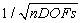for 2D problems, and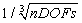for 3D problems, with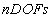the total number of degrees of freedom per equation, unless otherwise specified.
• For steady problems, start your computation from a uniform free-stream unless otherwise specified. Use the L2 norm of the density residual to monitor convergence. Steady state is assumed if the initial residual is dropped by 10 orders of magnitude. For cases impossible to converge 10 orders, 8 orders can be used as a convergence criterion. For the flat plate boundary layer problem, use the L2 norm of the x-momentum residual to monitor convergence.
• For each p (order of accuracy), compute the work units for 100 residual evaluations with 250,000 degrees-of-freedom per equation. Use your finest mesh, and scale your results for 250,000 DOFs. Submit the results to the workshop e-mail address.
• Results submission: If you compute more than one case, submit your results using separate messages. Put “Case CX.X Results” in your subject line. Submit all results to: hiocfd@gmail.com.
• Computational meshes: The gmsh format (http://www.geuz.org/gmsh/doc/texinfo/gmsh.html) is adopted for the workshop. The latest user’s manual is also here. Computational meshes in the same refinement sequence are solicited from the participants (but not required to participate). Good meshes will be posted on the web site to serve as common meshes for all participants.

If you generate new meshes, please adhere to the following guideline: For all 2d external flow problems, the far field should be a circle, centered at the airfoil mid chord (or the 1st airfoil) with a radius of 1000 chords. Do not apply any vortex correction at the far field.
• Residual definition for convergence monitoring: It is not trivial to define a residual easily computable for all methods. Consider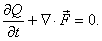The integration of the equation on element Vi is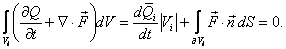Now replacing the normal flux term with any Riemann flux as the numerical flux, we obtain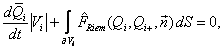where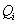is the reconstructed approximate solution on Vi, and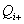is the solution outside Vi. The element residual is defined as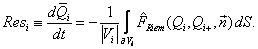Then the L2 norm of the residual is defined as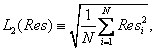where N is the total number of elements or cells. For a node based finite difference method, it is ok to use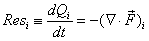as the residual definition. These two definitions are expected to differ by a second order term.

Furthermore, note that the definition of Res_i above is an example only. For different equations and different discretizations considered the definition of Res_i needs to be modified to coincide with the discretization-specific residual of the scheme.

• Error computation: For any solution variable (preferably non-dimensional) s, the L2 error is defined as (Option 1)For an element or cell based method (FV, DG etc), where a solution distribution is available on the element, the element integral should be computed with a quadrature formula of sufficient precision, such that the error is nearly independent (with 3 significant digits) of the quadrature rule. Note that for a FV method, the reconstructed solution should be the same as that used in the actual residual evaluation.

For a finite difference scheme, if the Jacobian matrix is available, i.e.,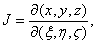the L2 error is defined as (Option 2a)Otherwise, the L2 error is defined as (Option 2b)For some numerical methods, an error defined based on the cell-averaged solution may reveal super-convergence properties. In such cases, we suggest another definition (Option 3a)In this definition, one can also drop the volume in a similar fashion to the definition for finite-difference type methods, i.e., (Option 3b)